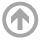Mathematics Department

# Combinatorics, Algebra, & Topology Seminar

## Spring 2020

All talks are from 1200-1300 in the designated room unless otherwise specified.

• Apr
20
• Anant Godbole
East Tennessee State University
Time: 12:00 PM
• Apr
09
• TBD
Xingting Wang
Howard University
Time: 12:00 PM
• Apr
02
• Cong X. Kang
Texas A&M University, Galveston
Time: 12:00 PM
• Mar
16
• Violeta Vasilevska
Utah Valley University
Time: 12:00 PM
• Feb
13
• Pebbling in Powers of Paths
Glenn Hurlbert
Virginia Commonwealth University
Time: 12:00 PM

#### View Abstract

The t-pebbling number of a graph G is defined to be the minimum number m so that, from any initial configuration of m pebbles on the vertices of G, it is possible to place at least t pebbles on any specified vertex via pebbling moves. It has been conjectured that the pebbling numbers of pyramid-free chordal graphs can be calculated in polynomial time. The kth-power G(k) of the graph G is obtained from G by adding an edge between any two vertices of distance at most k from each other. The kth-power of the path P_n on n is an important class of pyramid-free chordal graphs, and is a stepping stone to the more general class of k-paths and the still more general class of interval graphs. Pachter, Snevily, and Voxman (1995) calculated π(P_n^(2)), Kim (2004) calculated π(P_n^(3)), and Kim and Kim (2010) calculated π(P_n^(4)). Recently, Alcón and I have calculated π_t(P_n^(k)) for all n, k, and t. The pebbling exponent e_π(G) of a graph G was defined by Pachter, et al., to be the minimum k for which π(G(k)) = n(G(k)). Of course, e_π(G) ≤ diam(G), and Czygrinow, Kierstead, Trotter, and I (2002) proved that almost all graphs G have e_π(G) = 1. Lourdusamy and Mathivanan (2015) proved several results on π_t(Cn2), and I proved (2017) an asymptotically tight formula for e_\pi(C ). Our formula for π_t(P(k)) allows us to to compute e_π(Pn) exactly.
• Jan
13
• Algebraically Defined Graphs in Two and Three Dimensions
Brian Kronenthal
Kutztown University

#### View Abstract

Abstract: In this talk, we will discuss algebraically defined bipartite graphs. Indeed, let F denote a field, and consider the bipartite graph whose partite sets P and L are copies of F^3 such that (p1, p2, p3) ∈ P and [q1, q2, q3] ∈ L are adjacent if and only if p2 + q2 = p1q1 and p3 + f3 = p1q1^2. This graph has girth eight, and of particular interest is whether it is possible to alter these equations by replacing p1q1 and p1q1^2 with other bivariate polynomials to create a nonisomorphic girth eight graph. In addition to discussing some results related to this question, as well as a two-dimensional analogue, we will also explain the connection between algebraically defined graphs and incidence geometry, which partially motivates this line of inquiry.

#### ARCHIVES